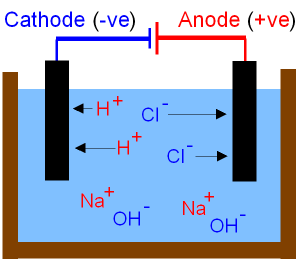gcsescience.com                                       12                                       gcsescience.com

The Periodic Table

What is an Aqueous Solution?

When a substance is
dissolved in water it is called an aqueous solution.

What are the
Products of the
Electrolysis of Aqueous Solutions?

The electrolysis of an aqueous solution can
give products from the ions in water rather than the
dissolved substance. Water is a compound
made from the elements hydrogen and oxygen.

We can predict which products will be discharged from
the electrodes by looking at the reactivity series.

If the metal is above hydrogen in the reactivity series then
hydrogen gas is discharged at the cathode. If the
metal is below hydrogen then the metal is discharged.

The table below shows the results of the electrolysis
of some substances that are dissolved in water.

 Dissolved Substance Formula Cathode Product Anode Product Copper Chloride CuCl2(aq) Copper Chlorine Cl2(g) Copper Sulfate CuSO4(aq) Copper Oxygen O2(g) Sodium Chloride NaCl(aq) Hydrogen H2(g) Chlorine Cl2(g) Sodium Sulfate Na2SO4(aq) Hydrogen H2(g) Oxygen O2(g) Hydrochloric Acid HCl(aq) Hydrogen H2(g) Chlorine Cl2(g) Sulfuric Acid H2SO4(aq) Hydrogen H2(g) Oxygen O2(g)

The Electrolysis of Sodium Chloride in Water.

See the previous page
for more detail of the electrolysis of sodium chloride in water.Question.
If water is a covalent molecule, where have the H+ hydrogen ions
and OH- hydroxide ions come from in the picture above?

The ionisation of water makes hydrogen ions and hydroxide ions.
Although hydrogen ions are only present in small amounts the
reversible ionisation means that the hydrogen ions are instantly
replaced when they are discharged at the cathode as hydrogen gas.
The water never runs out of hydrogen ions and hydroxide ions.

Question
.
Why don't the Na+ sodium ions and hydroxide ions
get discharged at the electrodes?

The (-)cathode attracts both Na+ sodium ions and H+ hydrogen ions.
Only one type of ion at a time can be discharged at the electrode.
The ion that is lower in the reactivity series is discharged first.
Hydrogen gas is discharged leaving the sodium ions in solution.

The (+)anode attracts both Cl- chloride ions and OH- hydroxide ions.
Chlorine gas is discharged leaving the hydroxide ions in solution.

The solution contains both sodium ions and hydroxide ions
and therefore becomes sodium hydroxide(aq).

See the uses of hydrogen,
the uses of chlorine and also the uses of sodium hydroxide.

gcsescience.com    The Periodic Table    Index    Periodic Table Quiz    gcsescience.com